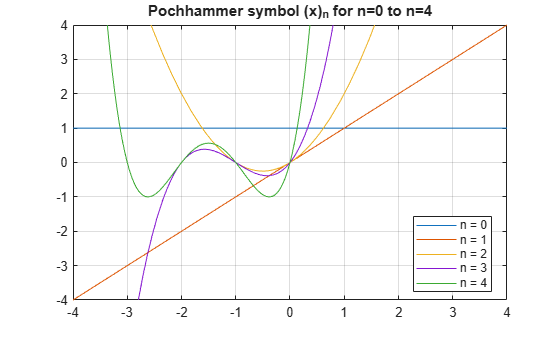# pochhammer

Pochhammer symbol

## Syntax

``pochhammer(x,n)``

## Description

example

````pochhammer(x,n)` returns the Pochhammer Symbol (x)n.```

## Examples

### Find Pochhammer Symbol for Numeric and Symbolic Inputs

Find the Pochhammer symbol for the numeric inputs `x = 3` at `n = 2`.

`pochhammer(3,2)`
```ans = 12```

Find the Pochhammer symbol for the symbolic input `x` at ```n = 3```. The `pochhammer` function does not automatically return the expanded form of the expression. Use `expand` to force `pochhammer` to return the form of the expanded expression.

```syms x P = pochhammer(x, 3) P = expand(P)```
```P = pochhammer(x, 3) P = x^3 + 3*x^2 + 2*x```

### Rewrite and Factor Outputs of Pochhammer

If conditions are satisfied, `expand` rewrites the solution using `gamma`.

```syms n x assume(x>0) assume(n>0) P = pochhammer(x, n); P = expand(P)```
```P = gamma(n + x)/gamma(x)```

To use the variables in further computations, clear their assumptions by recreating them using `syms`.

`syms n x`

To convert expanded output of `pochhammer` into its factors, use `factor`.

```P = expand(pochhammer(x, 4)); P = factor(P)```
```P = [ x, x + 3, x + 2, x + 1]```

### Differentiate Pochhammer Symbol

Differentiate `pochhammer` once with respect to `x`.

```syms n x diff(pochhammer(x,n),x)```
```ans = pochhammer(x, n)*(psi(n + x) - psi(x))```

Differentiate `pochhammer` twice with respect to `n`.

`diff(pochhammer(x,n),n,2)`
```ans = pochhammer(x, n)*psi(n + x)^2 + pochhammer(x, n)*psi(1, n + x)```

### Taylor Series Expansion of Pochhammer Symbol

Use `taylor` to find the Taylor series expansion of `pochhammer` with ```n = 3``` around the expansion point `x = 2`.

```syms x taylor(pochhammer(x,3),x,2)```
```ans = 26*x + 9*(x - 2)^2 + (x - 2)^3 - 28```

### Plot Pochhammer Symbol

Plot the Pochhammer symbol from `n = 0` to `n = 4` for `x`. Use `axis` to display the region of interest.

```syms x fplot(pochhammer(x,0:4)) axis([-4 4 -4 4]) grid on legend('n = 0','n = 1','n = 2','n = 3','n = 4','Location','Best') title('Pochhammer symbol (x)_n for n=0 to n=4')```## Input Arguments

collapse all

Input, specified as a number, vector, matrix, or multidimensional array, or a symbolic number, variable, vector, matrix, multidimensional array, function, or expression.

Input, specified as a number, vector, matrix, or multidimensional array, or a symbolic number, variable, vector, matrix, multidimensional array, function, or expression.

collapse all

### Pochhammer Symbol

Pochhammer’s symbol is defined as

`${\left(x\right)}_{n}=\frac{\Gamma \left(x+n\right)}{\Gamma \left(x\right)},$`

where Γ is the Gamma function.

If n is a positive integer, Pochhammer’s symbol is

`${\left(x\right)}_{n}=x\left(x+1\right)...\left(x+n-1\right)$`

## Algorithms

• If `x` and `n` are numerical values, then an explicit numerical result is returned. Otherwise, a symbolic function call is returned.

• If both `x` and `x + n` are nonpositive integers, then

`${\left(x\right)}_{n}={\left(-1\right)}^{n}\frac{\Gamma \left(1-x\right)}{\Gamma \left(1-x-n\right)}.$`

• The following special cases are implemented.

`$\begin{array}{l}{\left(x\right)}_{0}=1\\ {\left(x\right)}_{1}=x\\ {\left(x\right)}_{-1}=\frac{1}{x-1}\\ {\left(1\right)}_{n}=\Gamma \left(n+1\right)\\ {\left(2\right)}_{n}=\Gamma \left(n+2\right)\end{array}$`
• If `n` is a positive integer, then `expand(pochhammer(x,n))` returns the expanded polynomial $x\left(x+1\right)...\left(x+n-1\right)$.

• If `n` is not an integer, then `expand(pochhammer(x,n))` returns a representation in terms of `gamma`.

## Version History

Introduced in R2014b